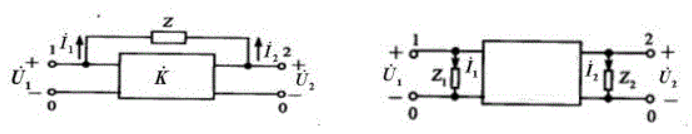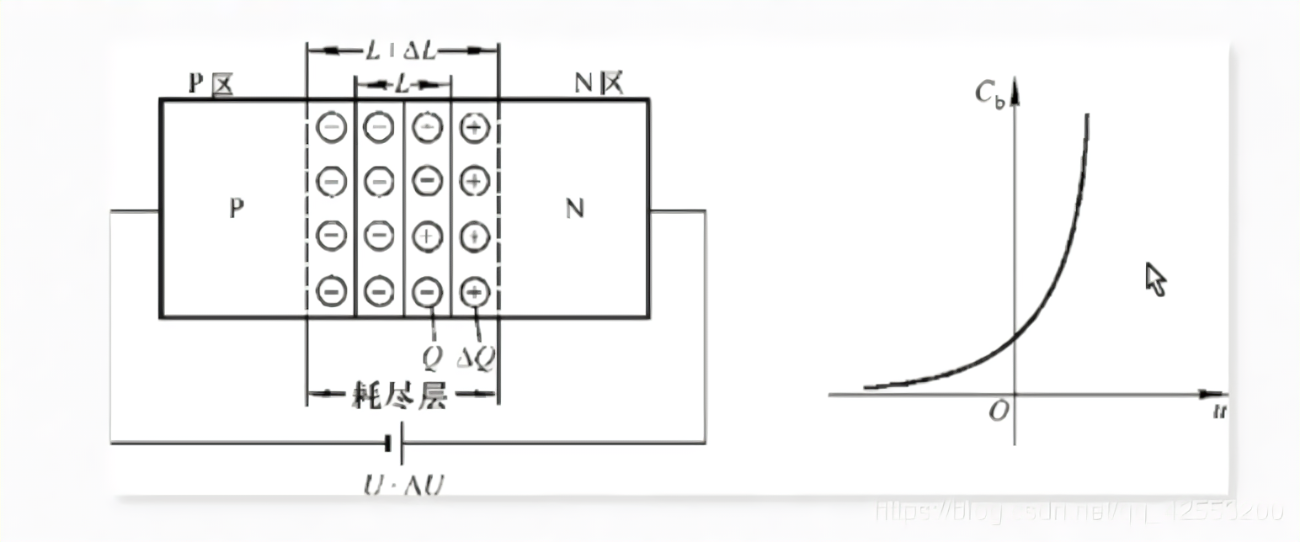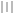# 半导体基础知识

## 1.1.0线性网络常用定理

• 柯希霍夫定律、叠加原理、戴维宁定理、诺顿定理等基础电路知识本章不再赘述，读者若不会或忘却，可自行查阅相关理论，下面只陈述之前未曾涉及的常用定理

### 米勒定理

• 对于有公共端的线性网络，跨接在A、B两点间的阻抗Z可分别等效为A，B对地的阻抗$Z_A$,$Z_B$
\begin{aligned} Z_A=\frac{Z}{1-\frac{V_B}{V_A}} \end{aligned}
\begin{aligned} Z_B=\frac{Z}{1-\frac{V_A}{V_B}} \end{aligned}
其中$V_A$,$V_B$分别是A和B对地的电压简单证明如下，我们设流入阻抗Z的电流为$I_1$，流入线性网络K的电流为$I_2$
对于左侧图
\begin{aligned} I=I_1+I_2=\frac{U_1-U_2}{Z}+I_2 \end{aligned}
对于右侧图
\begin{aligned} I=\frac{U_1}{Z_1}+I_2 \end{aligned}
联合得到
\begin{aligned} Z_1=\frac{Z}{1-\frac{U_2}{U_1}} \end{aligned}
同理可以得到$Z_2$

### 米勒对偶定理

• 米勒对偶定理是一个阻抗等效定理
• 在串联回路中，如果一个元件上的电流是输入端电流的K倍，则从输入端视入，该元件的扽报销阻抗也是原值的K倍
• 我们此后在晶体管的讲解中将广泛运用米勒定理的结论

## 1.1.1本征半导体

• 纯净的具有晶体结构的半导体称为本征半导体

### 半导体

• 常用的半导体材料为硅和锗，他们均为四价元素.
• 在形成晶体结构的半导体，人为掺杂特定元素时，导电性能具有可控性；并且在光照和辐射条件下，其导电性能还有明显的变化.

### 本征半导体的晶体结构

• 晶体中的原子在空间中形成排列整齐的点阵，称为晶格
• 相邻原子共用电子，形成共价键

### 本征半导体中的载流子

• 价电子由于热运动(热激发)获得足够能量后，挣脱共价键的束缚变成自由电子，此时在共价键的位置上形成一个空位置，称作空穴, 空穴带正电.
• 在本征半导体中，自由电子和空穴是成对存在的.
• 若在本征半导体两端加载一外电场，一方面自由电子将产生定向移动，形成电子电流；另一方面，由于空穴存在，价电子将按一定方向依次填补空穴，也就是说空穴也产生定向移动，形成空穴电流.由于空穴和自由电子的带电性质相反，因此两股电流的传导方向相反，本征半导体中的电流是空穴电流和电子电流之和.
• 运载电荷的粒子称为载流子，导体导电中只有自由电子导电；而本征半导体中有两种载流子，即自由电子和空穴均参与导电.

### 本征半导体中的载流子浓度

• 半导体在热激发下产生的自由电子和空穴对的现象称为本征激发
• 自由电子在运动过程中与空穴相遇就会填补空穴，这种现象称为复合
• 在一定温度下，本征半导体中的载流子浓度是一定的，并且自由电子浓度与空穴浓度相等.
• 本征半导体中的载流子浓度与温度密切相关.温度升高时，半导体中的载流子浓度上升，导电性能增强.温度降低则相反.实验分析表明本征半导体中的载流子浓度是温度的函数：
\begin{aligned} n_i = p_i = K_1 T^{\frac{3}{2}}e^{\frac{-E_{CO}}{2kT}} \end{aligned} \tag{1.1.1}
式$(1.1.1)$中的$n_i 和 p_i$分别表示自由电子与空穴的浓度($cm^{-3}$)，T是热力学温度，k是玻尔兹曼常数($8.63 \times10^{-5} eV/K$)，$E_{CO}$为热力学零度时破坏共价键所需的能量，又称为禁带宽度，$K_1$是与半导体材料载流子有效质量、有效能级密度有关的常量.

## 1.1.2杂质半导体

• 在本征半导体中掺入少量合适的杂质元素，便可以得到杂质半导体
根据掺入杂质元素不同，可以形成N型半导体和P型半导体

### N型半导体

• 在纯净的硅晶体中掺入五价元素(比如磷)，使之取代晶格中硅原子的位置，就形成了N型半导体
• 由于五价原子多出一个价电子，因此，在N型半导体中自由电子的浓度大于空穴浓度，故称自由电子为多数载流子(多子)，空穴为少数载流子(少子)，由于掺杂原子可以提供多余电子，因此称为施主原子，N型半导体主要靠自由电子导电.

### P型半导体

• 在纯净硅中掺杂三价元素(比如硼)，使之取代晶格中硅的位置，形成P型半导体.
• 由于三价元素缺少一个价电子，因此在形成共价键的时候就会多出一个空位，当硅原子的价电子前来填补空位时，在原有的共价键处就会形成一个空穴，因此在P型半导体中，空穴是多子，自由电子是少子，P型半导体主要依靠空穴导电.杂志原子称为受主原子

• 对于P、N型杂质半导体，多子的浓度越高，少子的宁都就越低.可以认为，多子浓度约等于所掺杂杂质原子的浓度，因而它受温度的影响很小.而少子是本征激发形成的，所以尽管浓度很低，但是受温度影响很高.
• 一句话就是：多子受温度影响低，少子受温度影响高

## 1.1.3 PN结

### PN结

• 物质总是从浓度高的地方向浓度低的地方扩散，这种由于浓度差产生的运动称为扩散运动
• 当把P型和N型半导体制作在一起时，由于两种类型的半导体主体载流子浓度差别很大，因此会产生扩散运动，从而出现大量复合，在交界面处的多子浓度就会下降.此时在P型半导体中就会出现负离子区，在N型半导体中就会出现正离子区，他们是不能移动的，称为空间电荷区，产生内电场.随着扩散运动的进行，空间电荷区区域加深，内电场增强，方向由N指向P，阻止扩散运动进行.形成的内电场称为内建场
• 由于内建场的存在，在电场力的作用下，载流子产生漂移运动，也即：内建电场是产生漂流运动的原因在空间电荷区形成后，少子产生漂移运动，空穴从N->P运动，自由电子从P->N运动.
• 在无外加电场的作用下，参与扩散运动的多子数目与少子数目相等，达到动态平衡，形成PN结.此时，空间电荷区域有一定的宽度，电位差为$U_{ho}$，电流为零.
• 理论分析表明，不加外电压时，PN结的内建电动势为：
\begin{aligned} \phi_0 = V_t(\frac{N_AN_D}{n^2_i}) \end{aligned}
其中$N_A,N_B,n_i$分别是受主浓度，施主浓度和本征浓度
$V_t$是热电势，也就是下面描述PN结电流方程里的$U_T$,二者是一样的:
\begin{aligned} V_t = U_T = \frac{kT}{q} \end{aligned}
• 以后我们取硅PN结的内建势为0.6V，室温下V_t = U_T =26mV*
• 当P、N型半导体内的杂志浓度相等时，形成的空间电荷区在两端是对称的，也称为对称结，杂质浓度不相等时称为不对称PN结，两种PN结的外部性质相同.
• 绝大部分空间电荷区内的空穴和自由电子都非常少，因此在分析PN结特性时常忽略载流子的作用，而只考虑离子区的电荷，这种方法称为耗尽层近似，因此空间电荷区也称为耗尽层

### PN结的单向导电性

• PN结两端外加电压时就会破坏原来的平衡状态，此时扩散电流就不再等于漂移电流.当外加电压极性不同时，PN结会表现出不同的导电性能，即呈现出单向导通性.
1. PN结外加正向电压处于导通状态
• 当电源正极或者正极串联电阻后连接到PN结的P端，且电源负极或负极串联电阻后接到PN结的N端时，称PN结外加正向电压，也称正向接法或正向偏置.
• 此时外加电场将多数载流子推向空间电荷区，使空间电荷区变窄，削弱内电场，破坏原来平衡，使得扩散运动加剧，漂移运动削弱，从而形成正向电流，PN结导通.
2. PN结外加反向电压时处于截止状态
• 当电源正极或者正极串联电阻后连接到PN结的N端，且电源负极或负极串联电阻后接到PN结的P端时，称PN结外加反向电压，也称反向接法或反向偏置.
• 外加电场使得空间电荷区变宽，加强内电场，阻止扩散运动进行，加剧漂移运动进行，形成反向电流，也称漂移电流
• 由于少子的数目极少，因此形成的反向电流很小，在分析时常常忽略不计，因此外反向电压时PN结处于截止状态.

### PN结的电流方程

• 理论分析可以得到，PN结两端所加电压u与流过它的电流i的关系为：
\begin{aligned} i = I_S(e^{\frac{qu}{kT}} - 1) \end{aligned} \tag{1.1.2}
式$(1.1.2)$中$I_S$为反向饱和电流，q为元电荷(即电子的电量)，k为玻尔兹曼常数，T为热力学温度.
• 定义$U_T = \frac{kT}{q}$,那么：
\begin{aligned} i = I_S(e^{\frac{u}{U_T}} - 1) \end{aligned} \tag{1.1.3}

### PN结的伏安特性

• 根据$(1.1.3)$，当PN结外加载正向电压，并且u >> $U_T$时，i ≈ $I_S(e^{\frac{u}{U_T}} )$，即i随u按照指数规律变化；当PN结加载反向电压，并且数值上u >> $U_T$时，i ≈ -$I_S$.
• 当反向电压超过一定数值时，反向电流急剧增加，称之为反向击穿.
• 击穿按照机理分为齐纳击穿雪崩击穿两种情况
• 齐纳击穿
在高掺杂的情况下，由于耗尽层的宽度很窄，不大的反向电压就可以在耗尽层形成很强的电场，可以直接破坏共价键，使得价电子脱离共价键约束，产生电子-空穴对，致使电流急剧增大，这种击穿称作齐纳击穿.
• 关于高掺杂情况下耗尽层宽度变窄而不是边宽的解释：
在高掺杂情况下，自由电子或空穴的浓度增大，因此扩散作用会增强，由此产生的内建电场强度会增大，耗尽层电压$U_{ho}$会增强，此时势垒电压会增大.但场强正相关于浓度，而势垒电压正向关于浓度的自然对数，根据U = Ed 可知间距会变窄.
• 雪崩击穿
在掺杂程度不大的情况下，耗尽层的宽度较宽，低反向电压无法产生齐纳击穿，而当反向电压增大到一定程度时，耗尽层内的电场使得少子加快漂移速度，从而在与共价键中的电子相碰撞过程中将价电子撞出共价键，产生空穴-自由电子对. 这样的过程是级联的，因为新产生的自由电子与空穴又参与撞击，致使电流急剧增加，这种击穿称为雪崩击穿### PN结的电容效应

• 在一定条件下，PN结具有电容效应，根据产生的原因不同可以将其分为势垒电容扩散电容
• 势垒电容
• 当加载在PN结两端的电压变化时，空间电荷区域的宽度会随之发生变化. .
• 耗尽层宽窄变化所引起的等效电容称之为**势垒电容$C_b$**，$C_b$具有非线性性，它与结面积、耗尽层深度、半导体介电常数以及外加电压有关.
• 势垒电容$C_b$由下述式子表示：
\begin{aligned} C_b = \frac{C_{b0}}{(1-\frac{V_D}{\varphi_0})^m} \end{aligned}
式中$\varphi_0$是内建势，m是常数(一般为1/3~1/2)，$V_D$是结电压，正偏为正，反偏为负，$C_{b0}$是在结电压为0时的势垒电容.• 扩散电容
• PN结处于平衡状态时的少子常称为平衡少子.PN结处于正向偏置时，从P扩散到N的空穴和从N扩散到P的自由电子称为非平衡少子.
• 在外加电压一定时，靠近耗尽区表面的地方非平衡少子的浓度高，而远离交界面的地方浓度低，并且浓度自高到低逐渐衰减到零，形成一定的浓度梯度，于是会形成扩散电流.当外加正向电压增大时，非平衡少子浓度增大并且浓度梯度也增大，因此扩散电流(正向电流)增大，反之相反.
• 扩散区内电荷的积累与释放与电容器充放电过程相同，这种电容效应称为扩散电容$C_d$.扩散电容具有非线性性，其与流过PN结的正向电流i、温度的电压当量$U_T(即\frac{kT}{q})$、非平衡少子的寿命$\tau$有关.
• $C_d = \alpha I_D$,可见反偏时扩散电容为零.
• 由此可见，PN结的电容$C_j是C_b与C_d之和$，低频信号时容抗很大，所以其作用可以忽略不计，因此我们只有在高频信号时才考虑结电容的作用.Invitation
USTC-茶糜花开
FeynmanDirac
created:12/03/2022
Welcome to USTC-茶糜花开

This is an identification card as an honored membership of FeynmanDirac

Happy to see you follow FeynmanDirac, enjoy science together验证码启动中...